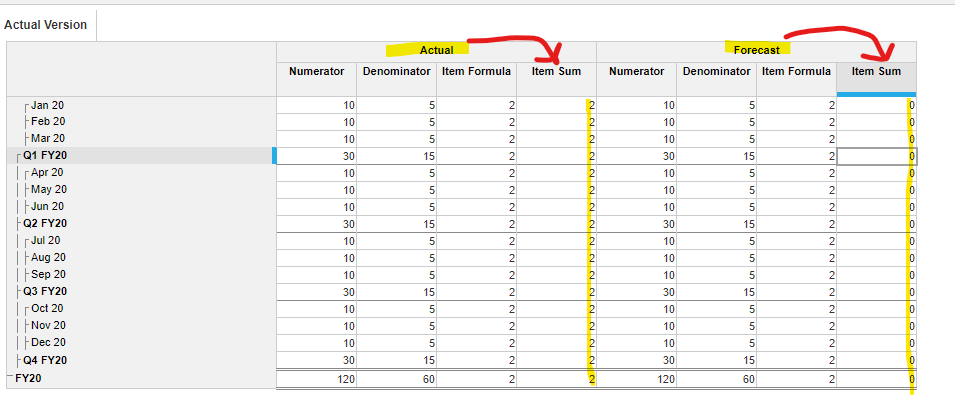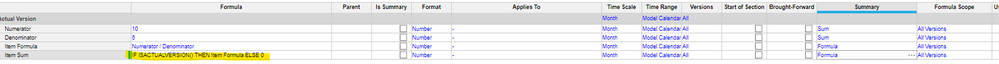# Formula Summary Method for Formula Scope set to Actuals OnlyHello All,

I am having trouble getting my summary method to work correctly. I have a line item that is set to formula scope 'Actual Version'(this cannot change), but I would like to use the summary method 'Formula'. Currently when trying to use the summary method formula I get the following error message "invalid combination of empty Formula, and summary set to Formula". I'm assuming this is because the module applies to all versions, but line item technically only has a formula for actual version, where all other versions are inputtable. Because of this limitation I have turned off the summary method to none because sum and average (the other summary method options) dont make sense when adding up percentages.

Does anyone have a work around for this? being able to use the formula summary when a line items formula needs to only apply to actuals?

Appreciate any feedback! thanks!

Tagged:

•Finally got around to this question. A good one I might add!

The version scope will dictate whether or not you can change the summary on that line item. If you chose "Actual Version" then you cannot use a "formula" summary because Anaplan won't know how you meant to summarize the non-actual versions.

So, here's are three ideas. Really depends on your use case so hopefully one of these will work for you.

1. Change the version scope to all versions. This will allow you to use any summary method applicable to the format of the line item.
2. Add another line item using the ISACTUALVERSION() formula and use a Boolean to determine which version you are using. Based on that logic you will compute the formula or not.3. Use a line item subset. If you go this route you can apply a formula to any version. This is a fairly unknown option. You can see it if you click on "Edit" in the blueprint menu and at the bottom you'll see "add version formula".

•A difficulty in summary options of % as with all ratios is that they do not sum up hierarchies or across time simply.

Therefore, I tend to try and always use ratio as my summary option when dealing % or any other ratio measure.

When using ratio you need to pull in both sides of the calculation but crucially you can use the results of both actuals and other versions to feed the summary calculation.

Therefore, the result of the actual formula and input can be incorporated into the summary calculation.

However, what you need to be careful of here is that the calculation will take the summary level of the inputs when calculating the totals of all levels in the hierarchy and across time.

Hopefully this can help you in working out the solution to this particular challenge.

Good luck,

Chris